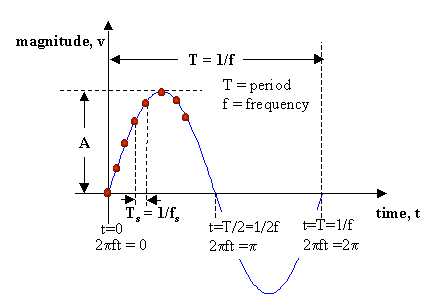# Networks I

##Making Music with MATLABBefore we actually start making music, let's revise a few AC waveform basics. Consider the sine wave shown in the figure below:The sine wave shown here can be described mathematically as:
v = A sin 2 p f t
where A is the Amplitude (varying units), f is the frequency (Hertz) and t is the time (seconds).
T is known as the time period (seconds) and T=1/f
Based on the equation,
when t=0; v = A sin 2 p f(0) = 0
when t=T/4; v = A sin 2 p f (T/4) = A sin 2 p f(1/4f) = A sin ( p/2) = A;
when t=T/2; v = A sin 2 p f (T/2) = A sin 2 p f(1/2f) = A sin ( p) = 0;
and so on.Sound waves are created when a waveform as shown here is used to vibrate molecules in a material medium at audio frequencies (300 Hz <= f <= 3 kHz).
If you wish to create a waveform such as the one shown in the figure, you would need to evaluate the function, v = A sin 2 p f t, at discrete times, t (shown as the time instants of the red dots), typically at equal time intervals. These time intervals must be "sufficiently" small (how small is small? Remember the Nyquist criterion?..........) to obtain a "smooth" waveform.
This time interval is called the "Sampling Interval" and the process of breaking up a continuous waveform by specifying it only at discrete points in time is called sampling. (you should already know much of this stuff from the previous Clinic on Data Acquisition Basics).
The sampling interval, shown here is Ts; corresponding to that there exists a "Sampling Frequency", fs = 1/Ts.As an example, the MATLAB code to create a sine wave of amplitude A = 1, at audio frequency of 466.16 Hz (corresponds to A# in the Equal Tempered Chromatic Scale) would be:

>> v = sin(2*pi*466.16*[0:0.000125:1.0]);

The vector, v, now contains samples of the sine wave, starting at t=0 s, to t=1.0 s, in samples spaced 0.000125 s apart (the sampling interval Ts). This corresponds to a sampling frequency of 8 KHz, which is standard for voice grade audio channel.

Now, you can either plot this sine wave; or you can hear it!!!

To plot, simply do
>> plot(v);

To hear v, you need to convert the data contained in v to some standard audio format that can be played using a Sound Card and Speakers on your PC. One such standard audio format is called the "wav" format. Matlab provides a function called wavwrite to convert a vector into wav format and save it on disk. Do >> help wavwrite for more details. Now, to create a wav file, do
>> wavwrite(v, 'asharp.wav'); (you can give any file name between the '  ' s)

Now, you can "play" this wav file called asharp.wav using any multimedia player. For example, you could use the Sound Recorder program. Start this by pointing to Start - Programs - Accessories - Multimedia - Sound Recorder. Open the wav file using the File Menu and press Play. If you have installed a sound card and a pair of speakers on your PC, you should be able to hear a short note.Now that you can make a single note, you can put notes together and make music!!!

Let's look at the following piece of music:
A A    E E    F# F#    E E
D D    C#C#    B B    A A
E E    D D    C# C#    B B (repeat once)
(repeat first two lines once)

The American Standard Pitch for each of this notes is:
A:    440.00 Hz
B:    493.88 Hz
C#:  554.37 Hz
D:    587.33 Hz
E:    659.26 Hz
F#:  739.99 Hz

Assuming each note lasts for 0.5 seconds, the MATLAB m-file for creating a wav file for this piece of music would be:

clear;
a=sin(2*pi*440*(0:0.000125:0.5));
b=sin(2*pi*493.88*(0:0.000125:0.5));
cs=sin(2*pi*554.37*(0:0.000125:0.5));
d=sin(2*pi*587.33*(0:0.000125:0.5));
e=sin(2*pi*659.26*(0:0.000125:0.5));
fs=sin(2*pi*739.99*(0:0.000125:0.5));

line1=[a,a,e,e,fs,fs,e,e,];
line2=[d,d,cs,cs,b,b,a,a,];
line3=[e,e,d,d,cs,cs,b,b];

song=[line1,line2,line3,line3,line1,line2];

wavwrite(song,'song.wav');

HINT: Before you code the entire song, just code the first line, create a wav file, play it and make sure everything works.

Do you recognize this piece? (If not, then we have a lot of work ahead of us..............) Enjoy!!!!!Calling All Musicians in the Class:
If you have notes for other songs; you can code them too. Also, using Sound Recorder, you can create harmonics, synthesize chords, etc. after generating the pure notes in Matlab.

Back to Course Homepage